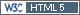## compromised power [Power / Sample Size]

Hi Darya,

» The sample size by the formula I used:
» n=28

To keep everything equal you should convert the standard deviation to the CV. Hence,

```library(PowerTOST) sampleN.TOST(CV=se2CV(0.3)) +++++++++++ Equivalence test - TOST +++++++++++             Sample size estimation ----------------------------------------------- Study design:  2x2 crossover log-transformed data (multiplicative model) alpha = 0.05, target power = 0.8 BE margins = 0.8 ... 1.25 True ratio = 0.95,  CV = 0.3068783 Sample size (total)  n     power 42   0.818541```

Of course higher than the 28 by the dubious formula. As David mentioned above (quoting Zhang’s paper) power is compromised. Let’s check how much:

```power.TOST(CV=se2CV(0.3), n=28)  0.6250166```

Oops!

Cheers,
Helmut SchützThe quality of responses received is directly proportional to the quality of the question asked. ☼
Science QuotesIng. Helmut Schütz